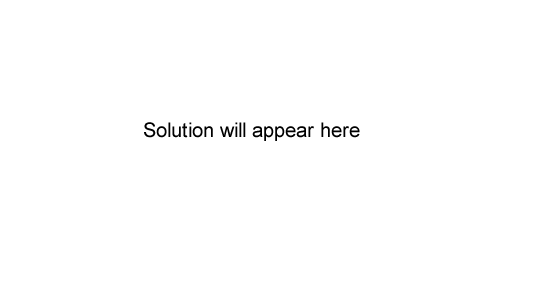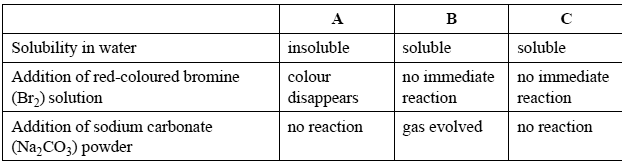Organic chemistry 2006 VCE Consider the molecule CH3CH2CH2CH2CHClCH2CH3. The systematic name of this molecule is A. 5-chloroheptane. B. 3-chloroheptane. C. 5-chlorooctane. D. 3-chlorooctane. SolutionThe raspberry- flavoured food additive, butyl methanoate, can be prepared from CH3CH2CH2CH2OH using A. an addition reaction with HCOOH. B. an addition reaction with CH3COOH. C. a condensation reaction with HCOOH. D. a condensation reaction with CH3COOH. SolutionThe number of structural isomers that are carboxylic acids with the formula C4H8O2 is A. 1 B. 2 C. 3 D. 4   SolutionTwo chemical reactions occur as follows. CH2=CH CH2CH2CH3 + X → 2-chloropentane and 2-chloropentane + sodium hydroxide solution → Y X and Y are given by A. X= Cl2 and Y= CH3CH2CH2CHOHCH3 B. X= HCl and Y= CH3CH2CH2CHOHCH3 C.X= Cl2 and Y= CH3CH2CH2CH2CH2OH D. X= HCl and Y= CH3CH2CH2CH2CH2OH SolutionA short section of a polymer molecule is shown below. CH2CF2CF2CH2CF2CH2CH2CF2CH2 This polymer could have been formed from A. CF2CH2 only. B. CF2CF2 and CH2CH2. C. CF2CF2 and CH2CHCF3. D. CH3CHCF2 and CF3CHCF2. SolutionThe molecule HOCH2CH2CH2COOH (molar mass = 104 g /mol) forms a polymer in which the average polymer molecule contains 1000 monomer units. The approximate molar mass of the polymer, in g/mol, is A. 68 000 B. 86 000 C. 95 000 D. 104 000 SolutionA student was given four colourless liquids that were labelled A, B, C and D. They were known to be ethanol, ethanoic acid, pentane and hexene, but the exact identity of each liquid was unknown. The student tested the properties of three of the liquids and obtained the results shown on the right. Identify each of the liquids. Solution